# Define Hooke’s law. Define three types of elastic modulus (Young’s Modulus, Bulk Modulus & Rigidity Modulus) with formulae?

9 years ago
Hooke's law states that the force F needed to extend or compress a spring by some distanceis proportional to that distance. That is:whereis a constant factor characteristic of the spring, its stiffness.

· Young's Modulus of elasticity

Iti It is defined as the ratio of normal stress to the longitudinal strain within elastic limit.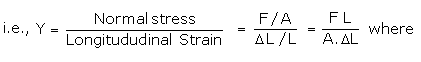F is the normal force applied at the end of its wire, A is area of cross section (= pr2), Dl is the extension produced due to normal force, L is the original length of the wire.

· Bulk Modulus of elasticity (K)

It is defined as the ratio of normal stress to the volumetric strain within the elastic limit.

Thus,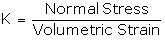· Consider a spherical solid body of volume V and surface area a, when a force F is applied normally, the volume decreases by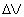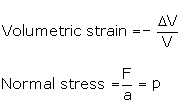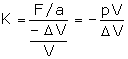Modulus of Rigidity

· It is defined as the ratio of tangential stress to the shearing strain within the elastic limit.

· Considering a solid metal cube whose lower face is fixed and its upper face is subjected to a tangential force F. The body suffers a change in its shape but not in its volume. If q is angle through which upper layer is sheared then,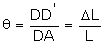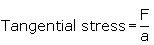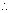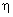(Modulus of Rigidity)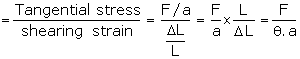Thanks & regard

Aarti Gupta

fcon